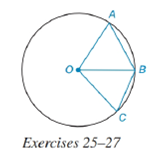Chapter 6.4, Problem 27EElementary Geometry For College St...

7th Edition
Alexander + 2 others
ISBN: 9781337614085

Solutions

Chapter
SectionElementary Geometry For College St...

7th Edition
Alexander + 2 others
ISBN: 9781337614085
Textbook ProblemIn ⊙ O , m ∠ A O B = 70 ° and m ∠ B O C = 30 ° . See the figure above.a) How are m A B ⌢ and m B C ⌢ related?b) How are A B and B C related?

To determine

(a)

To find:

The relation between mAB and mBC.

Explanation

Given:

O, mAOB=70° and mBOC=30°. The figure given below

Theorem Used:

According to the following theorem

In a circle (or in congruent circles) containing two unequal central angles, the larger angle corresponds to the larger intercepted arc.

Calculation:

Using the theorem:

In a circle (or in congruent circles) containing two unequal central angles, the larger angle corresponds to the larger intercepted arc.

Since the angles mAOB=70° and mBOC=30° are unequal and mBOC<mAOB and from the given figure,

To determine

(b)

To find:

The relation between AB and BC.

Still sussing out bartleby?

Check out a sample textbook solution.

See a sample solution

The Solution to Your Study Problems

Bartleby provides explanations to thousands of textbook problems written by our experts, many with advanced degrees!

Get Started

In Exercises 4148, find the indicated limit given that limxaf(x)=3 and limxag(x)=4 41. limxa[f(x)g(x)]

Applied Calculus for the Managerial, Life, and Social Sciences: A Brief Approach

Evaluate the expression sin Exercises 116. 52535252

Finite Mathematics and Applied Calculus (MindTap Course List)

Subtract: 2301506

Elementary Technical Mathematics

True or False: The figure at the right is the graph of the direction field for y = (y 1)x2.

Study Guide for Stewart's Single Variable Calculus: Early Transcendentals, 8th

True or False: , for –π ≤ t ≤ π, is smooth.

Study Guide for Stewart's Multivariable Calculus, 8th